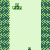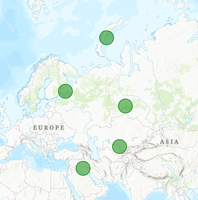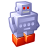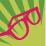# Creating Spatial Enabled Dataframe from pandas dataframe with Latitude and Longitude coordinates?

3982
9
03-18-2021 10:59 AMNew Contributor III

I have a pandas dataframe with latitude and longitude columns. I would like to convert it ultimately to a feature class. In the conversion to a spatial data frame, I do the following.

sdf = arcgis.features.GeoAccessor.from_xy(dff, x_column='longitude', y_column='latitude', sr=4326)

I used this tutorial as a guide https://developers.arcgis.com/python/guide/part2-working-with-geometries/#Create-an-SeDF-object-with...

and keep getting this exception message

raise Exception("Spatial column not defined, please use `set_geometry`")

Exception: Spatial column not defined, please use `set_geometry`

1 Solution

Accepted SolutionsbyMVP Notable Contributor

Here we go.

#### The Standard Method

``````from arcgis.features import GeoAccessor
import pandas as pd
from numpy.random import rand

lats = rand(5) * 45 + 30
lons = rand(5) * 45 + 30

df = pd.DataFrame({'lat':lats, 'lon':lons})``````

Here's df:

``````|   |       lat |       lon |
|--:|----------:|----------:|
| 0 | 66.212195 | 42.930500 |
| 1 | 58.392814 | 74.032695 |
| 2 | 44.315964 | 32.602737 |
| 3 | 69.484491 | 58.170186 |
| 4 | 33.592844 | 62.363671 |``````

Using the standard from_xy seems to work as intended.

``GeoAccessor.from_xy(df, 'lon', 'lat').spatial.plot()``

Results in:This even works when the lat/lon values are null or clearly invalid ( > 180 ), it still "works", it just doesn't render correctly on the map.

#### Let's Pretend that Didn't Work: Alternate Method

I have had it happen where the normal conversion process just wouldn't work. To work around that, we'll wrangle the lat/lon values into a string of the same format that the SEDF uses.

``df['SHAPE'] = '"spatialReference": {"wkid": 4326}, {"x":' + df['lon'].astype('str') + ', "y":' + df['lat'].astype('str') + '}'``

This results in a new column 'SHAPE' with values like this:

`'{"spatialReference": {"wkid": 4326}, "x": 67.91584605411032, "y": 34.142203783078585 }`

Which you may recognize as the JSON text of point geometries, seen in SEDFs. Let's now try to assign this as the geometry column.

``````sdf = GeoAccessor.from_df(df, geometry_column='SHAPE')
sdf.spatial.plot()``````

Which results in (different iteration of script, so my random values have shifted):Give this alternate method a shot. If that doesn't work, you may be in a pickle. Or you may need to take a harder look at the input data.

- Josh Carlson
Kendall County GIS
9 RepliesbyMVP Notable Contributor

I don't see a reason why this wouldn't work, given your code.

Can you check dff.dtypes? I would guess that the problem may lie with the input dataframe.

- Josh Carlson
Kendall County GISNew Contributor III

here are the dtypes for longitude and latitude

longitude float64
latitude float64

The rest of the columns are listed as object.byMVP Notable Contributor

Here we go.

#### The Standard Method

``````from arcgis.features import GeoAccessor
import pandas as pd
from numpy.random import rand

lats = rand(5) * 45 + 30
lons = rand(5) * 45 + 30

df = pd.DataFrame({'lat':lats, 'lon':lons})``````

Here's df:

``````|   |       lat |       lon |
|--:|----------:|----------:|
| 0 | 66.212195 | 42.930500 |
| 1 | 58.392814 | 74.032695 |
| 2 | 44.315964 | 32.602737 |
| 3 | 69.484491 | 58.170186 |
| 4 | 33.592844 | 62.363671 |``````

Using the standard from_xy seems to work as intended.

``GeoAccessor.from_xy(df, 'lon', 'lat').spatial.plot()``

Results in:This even works when the lat/lon values are null or clearly invalid ( > 180 ), it still "works", it just doesn't render correctly on the map.

#### Let's Pretend that Didn't Work: Alternate Method

I have had it happen where the normal conversion process just wouldn't work. To work around that, we'll wrangle the lat/lon values into a string of the same format that the SEDF uses.

``df['SHAPE'] = '"spatialReference": {"wkid": 4326}, {"x":' + df['lon'].astype('str') + ', "y":' + df['lat'].astype('str') + '}'``

This results in a new column 'SHAPE' with values like this:

`'{"spatialReference": {"wkid": 4326}, "x": 67.91584605411032, "y": 34.142203783078585 }`

Which you may recognize as the JSON text of point geometries, seen in SEDFs. Let's now try to assign this as the geometry column.

``````sdf = GeoAccessor.from_df(df, geometry_column='SHAPE')
sdf.spatial.plot()``````

Which results in (different iteration of script, so my random values have shifted):Give this alternate method a shot. If that doesn't work, you may be in a pickle. Or you may need to take a harder look at the input data.

- Josh Carlson
Kendall County GISNew Contributor III

Wow thank you this worked perfectly!New Contributor

I tried implementing your code to convert the pandas dataframe to a spatially enabled dataframe. It looks like it almost works, in that, it automatically zoomed into the correct location where the points should be. However, the points are not actually appearing on the map. I've included the implemented code in the top cell, the output, as well as an example of the SEDF that I am trying to plot with the SHAPE column featured in the bottom cell. Any help would be greatly appreciated!New Contributor III

You can try to use GeoAccessor.set_geometry function to set the column(s) that contain the geometry.

``sdf = sdf.set_geometry(['longitude', 'latitude'])``

You can set the parameter "inplace = True". This will change the DF without returning a copy of it.New Contributor III

Thanks Mark for the Quick response I receive the following messages when I try the above code

ValueError: Can't do inplace setting when converting from DataFrame to SpatialDataFrame

if I point at another dataframe

TypeError: Input geometry column must contain valid geometry objects.

I guess I can go back to the tutorial and see what I am missing. Any further suggestions are greatly appreciated!

MichaelbyEsri Contributor

It's working for me too.

You may need to test another dataset and may also consider upgrading ArcGIS API for Python.byMVP Regular Contributor

umm... It should work.

It would be helpful for the diagnosis if we can see a snapshot of the DataFrame an exact code that is doing the conversion.

Btw, which version of ArcGIS API for Python are you using?